## Algorithms and Data Structures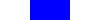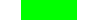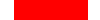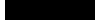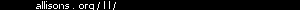LA home Computing Algorithms  glossary also see... Prog.Lang
 Code for some of the algorithms discussed exists in various programming languages such as Algol, C, Haskell, Java, Pascal and SML. The Bibliography also contains references on algorithms and data structures in journals and books.

Algorithm: a process or set of rules used for calculation or problem-solving, esp. with a computer.
Program: a series of coded instructions to control the operation of a computer or other machine. [-concise OED '91]

## Example

Problem: Find the greatest common divisor (GCD) of two integers, m and n.
Euclid's Algorithm:

```while m is greater than zero:
If n is greater than m,
swap m & n.
Subtract n from m.
n is the GCD
```
Program (in C):
```int gcd(int m, int n)
/* precondition: m>0 & n>0.
Let g=gcd(m,n). */
{ while( m > 0 )
{ /* invariant: gcd(m,n)=g */
if( n > m ) /* swap */
{ int t=m; m=n; n=t; }
/* m >= n > 0 */
m -= n;
}
return n;
}
```

The greatest common divisor (GCD) of [] & [] is [].
trace:

### Correctness

Why do we believe that this algorithm devised thousands of years ago, is correct?

Given m>0 and n>0, let g = gcd(m,n).
(i) If m=n then m=n=gcd(m,n) and the algorithm sets m to zero and returns n, obviously correct.
(ii) Otherwise, suppose m>n. Then m=p×g and n=q×g where p and q are coprime, from the definition of greatest common divisor. We claim that gcd(m-n,n)=g. Now m-n=p×g-q×g=(p-q)g. so we must show that (p-q) and q are coprime. If not then p-q=a×c and q=b×c for some a,b,c>1. But then p=q+a×c =b×c+a×c =(a+b)×c and because q=b×c, p and q would not have been coprime ... contradiction. Hence (p-q) and q are coprime, and gcd(m-n,n)=gcd(m,n).
(iii) If m<n, the algorithm swaps them so that m>n and that case has been covered.

So the algorithm is correct, provided that it terminates.

#### Termination

At the start of each iteration of the loop, either n>m or m≥n.
(i) If m≥n, then m is replaced by m-n which is smaller than the previous value of m, and still non-negative.
(ii) If n>m, m and n are exchanged, and at the next iteration case (i) will apply.
So at each iteration, max(m,n) either remains unchanged (for just one iteration) or it decreases.
This cannot go on for ever because m and n are integers (this fact is important), and eventually a lower limit is reached, when m=0 and n=g.

So the algorithm does terminate.

### Testing

Having proved the algorithm to be correct, one might argue that there is no need to test it. But there might be an error in the proof or maybe the program has been coded wrongly. Good test values would include:

• special cases where m or n equals 1, or
• m, or n, or both equal small primes 2, 3, 5, ..., or
• products of two small primes such as p1×p2 and p3×p2,
• some larger values, but ones where you know the answers,
• swapped values, (x,y) and (y,x), because gcd(m,n)=gcd(n,m).
The objective in testing is to "exercise" all paths through the code, in different combinations.

Debugging code be inserted to print the values of m and n at the end of each iteration to confirm that they behave as expected.

### Complexity

We are interested in how much time and space (computer memory) a computer algorithm uses; i.e. how efficient it is. This is called time- and space- complexity. Typically the complexity is a function of the values of the inputs and we would like to know what function. We can also consider the best-, average-, and worst-cases.

#### Time

The time to execute one iteration of the loop depends on whether m>n or not, but both cases take constant time: one test, a subtraction and 4 assignments v. one test, a subtraction and one assignment. So the time taken for one iteration of the loop is bounded by a constant. The real question then is, how many iterations take place? The answer depends on m and n.

If m=n, there is just one iteration; this is the best-case.
If n=1, there are m iterations; this is the worst-case (equivalently, if m=1 there are n iterations).
The average-case time-complexity of this algorithm is difficult to analyse.

#### Space

The space-complexity of Euclid's algorithm is a constant, just space for three integers: m, n and t. We shall see later that this is 'O(1)'.

## Exercises

• Devise a quicker version of Euclid's algorithm that does not sit in the loop subtracting individual copies of n from m when m>>n.